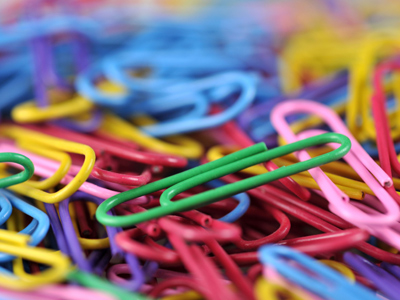You would use grams to measure the weight of a paper clip.

# Measures 4

This Math quiz is called 'Measures 4' and it has been written by teachers to help you if you are studying the subject at middle school. Playing educational quizzes is a fabulous way to learn if you are in the 6th, 7th or 8th grade - aged 11 to 14.

It costs only \$12.50 per month to play this quiz and over 3,500 others that help you with your school work. You can subscribe on the page at Join Us

Children should be quite comfortable when dealing with measures in Math. By now they should be well aware of the metric measures (mm, cm, m, km, g, kg, tons, ml, cl and l) and know some of the imperial measures (pint, gallon, mile etc.). They should also be able to convert smaller and larger units and combine the two (eg. 2.8 m = 280 cm = 2m 80cm). They will now be introduced to the imperial units of weight – ounces, pounds and tons, and how they relate to grams, kilograms and tons.

Tons, stones, pounds and ounces are all types of measures. They are all used to measure weight, but nowadays tons, kilograms and grams are more common. Babies used to be weighed in pounds and ounces when they were first born. These days, they are weighed in grams. Are you able to change from pounds and ounces to grams quickly and easily?

Which weight is highest - 1,000 kilograms or 1 ton? Find out in this measures quiz.

1.
How many ml in 1 centiliter?
1ml
10ml
100ml
1,000ml
1 liter = 100cl = 1,000ml
2.
Which unit would you use to measure the distance between the Moon and Earth?
cm
metres
mm
miles
Kilometers could also be used
3.
Roughly how many pounds in 1kg?
1 pound
2 pounds
2.2 pounds
2.5 pounds
1lb (1 pound) = 454g
4.
How do we write 375ml in liters?
0.375 l
3.75 l
37.5 l
375 l
To convert milliliters to liters, divide by 1,000
5.
How many kg in one ton?
10kg
100kg
1,000kg
10,000kg
A non-metric ton is equal to 1,016kg - slightly heavier than a metric ton
6.
What is 4.219km in meters?
42.19m
421.9m
4,219m
42,190m
To convert kilometers to meters, times by 1,000
7.
Which unit would you use to measure the weight of a paper clip?
kg
ml
cm
grams
Some paperclips would probably weigh even less than a gram!
8.
How many miles in 8 km?
0.5 mile
5 miles
50 miles
500 miles
To convert km to miles divide by 8 and then times by 5.
To convert miles to km divide by 5 and then times by 8
9.
Approximately how many grams are equal to 1 ounce?
0.3g
3g
30g
300g
1oz (1 ounce) = 28.3g
10.
100 centiliters is equal to?
1 liter
10 liters
100 liters
1,000 liters
We can shorten centiliter to cl
Author:  Amanda Swift